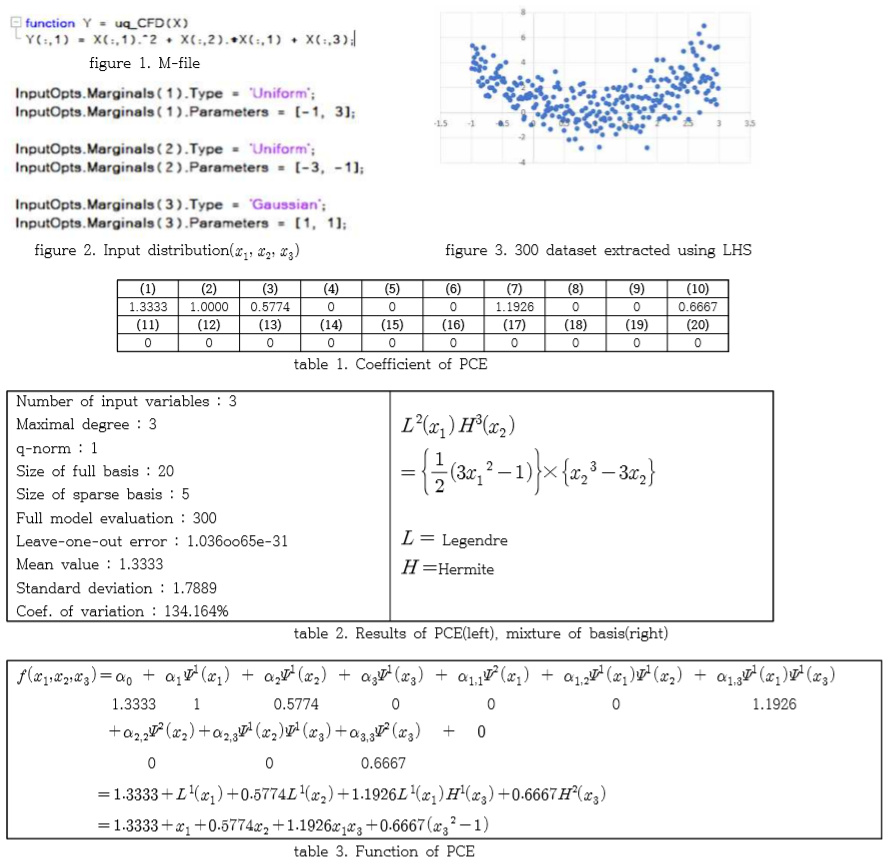# [Additional question of below question] PCE function extract

Hi, uqlabfirst of all, thanks to the developers who created the nice package.
After creating a data driven PCE model, i want to proceed with robust optimization after extracting the PCE function.Thus, an m-file was created to solve the toy problem as shown in figure 1. In addition, a total of three input variables were entered and the distribution was input as shown in figure 2.

Thus, a PCE model was created, and the results of the model are shown in Table 1 and Table 2.

Since the coefficient is 0 from the 11th to the 20th, there is no basis for the third order.
What I am trying to do is extract the PCE function and optimize it with MATLAB fmincon.

1. The PCE formula was referenced by referring to several papers. I wonder if it is right to expand in the above order(table 3).

0 1 2 3 [1,1] [1,2] [1,3] [2,2] [2,3] [3,3] …

When expand in the above order, it is different from the validation result of the model. Can i extract basis from uqlab package?

X_val = [1.7118, -2.8774, 1.9047] Y_val = [-0.0906], Y_cal = [7.024]

1. x1 and x2 are Legendre polynomial and x3 is Hermite polynomial. If Legendre and Hermite are multiplied, is it simply to multiply the two polynomials? (table 2. right)

2. What is the basis of arbitrary PCE? I would like to know if there are related literature.

I would be very grateful with your response.

Hi @Chemicaleng,

Thanks for the post.

1. As @nluethen has pointed out in another post, the basis functions you defined here corresponds to a standard random variable. For example, your Legendre polynomials L are mutually orthogonal with respect to the probability measure of the uniform distribution \mathcal{U}(-1,1), whereas the Hermite polynomials you defined form a set of basis with respect to the probability measure of the standard normal distribution \mathcal{N}(0,1). For a simple verification, you calculate some properties of the basis function in your table L^2(X_1) = \frac{1}{2}\left(3\,X_1^2 - 1\right): its expectation should be zero, which is not the case if you use X_1 \sim \mathcal{U}(-1,3).

2. Yes. As far as your input variables are independent, you can multiply the associated univariate basis functions to get multivariate bases.

3. In general, UQLab does not use the explicit form of the orthogonal polynomials. Instead, they are evaluated efficiently in a recursive way. For arbitrary PCEs, please refer to the PCE manual (Section 1.3.1.2, page 3) and also this book for details.